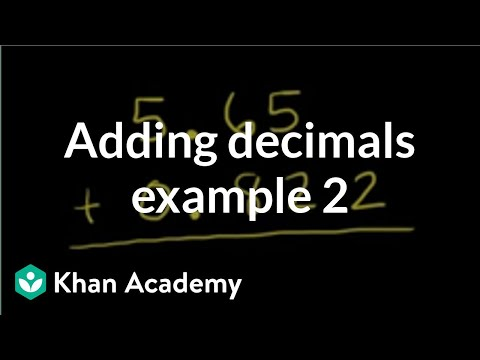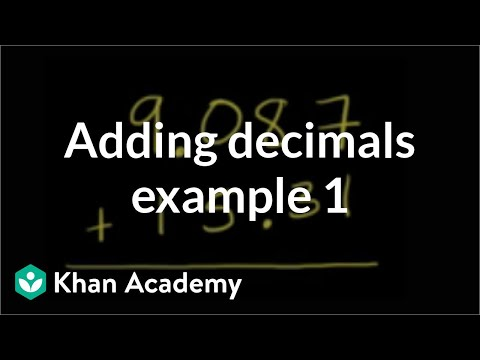Video

# Dividing decimals completely (Full video)

Description: Sal shows how to divide 6.3 by 0.25. Let's figure out what 6.3 divided by 0.25 is. And if I multiply it by 10 twice, then I'm gonna move two spots to the right. So let's see, if I move it to the right once, I get 63, so that's one multiplication by 10.

### Other videos you might be interested in### Adding decimals: 0.822 + 5.65 (Full video)